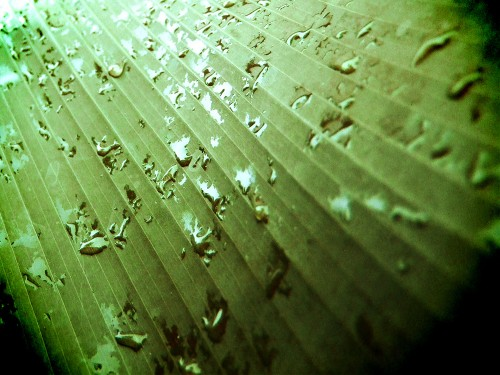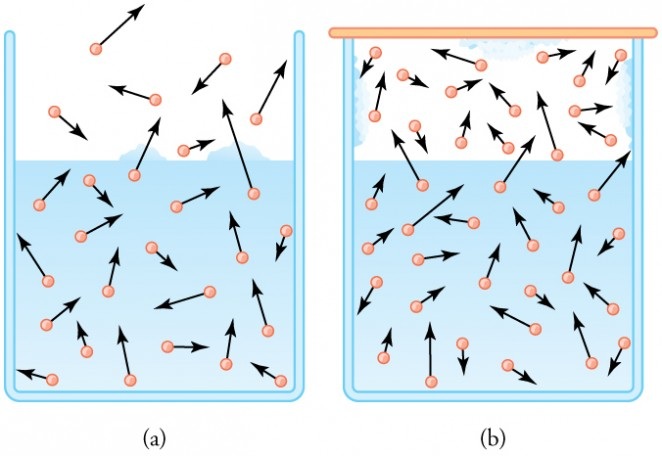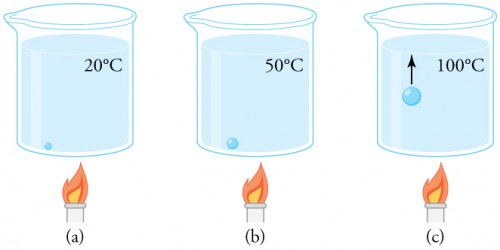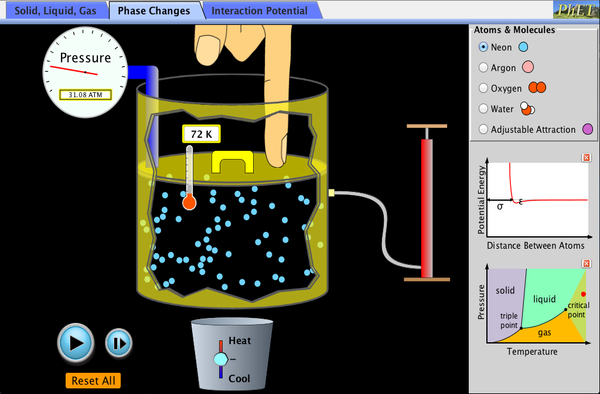## Humidity, Evaporation, and Boiling

### Learning Objectives

By the end of this section, you will be able to:

• Explain the relationship between vapor pressure of water and the capacity of air to hold water vapor.
• Explain the relationship between relative humidity and partial pressure of water vapor in the air.
• Calculate vapor density using vapor pressure.
• Calculate humidity and dew point.Figure 1. Dew drops like these, on a banana leaf photographed just after sunrise, form when the air temperature drops to or below the dew point. At the dew point, the air can no longer hold all of the water vapor it held at higher temperatures, and some of the water condenses to form droplets. (credit: Aaron Escobar, Flickr)

The expression “it’s not the heat, it’s the humidity” makes a valid point. We keep cool in hot weather by evaporating sweat from our skin and water from our breathing passages. Because evaporation is inhibited by high humidity, we feel hotter at a given temperature when the humidity is high. Low humidity, on the other hand, can cause discomfort from excessive drying of mucous membranes and can lead to an increased risk of respiratory infections.

When we say humidity, we really mean relative humidity. Relative humidity tells us how much water vapor is in the air compared with the maximum possible. At its maximum, denoted as saturation, the relative humidity is 100%, and evaporation is inhibited. The amount of water vapor the air can hold depends on its temperature. For example, relative humidity rises in the evening, as air temperature declines, sometimes reaching the dew point. At the dew point temperature, relative humidity is 100%, and fog may result from the condensation of water droplets if they are small enough to stay in suspension. Conversely, if you wish to dry something (perhaps your hair), it is more effective to blow hot air over it rather than cold air, because, among other things, hot air can hold more water vapor.

The capacity of air to hold water vapor is based on vapor pressure of water. The liquid and solid phases are continuously giving off vapor because some of the molecules have high enough speeds to enter the gas phase; see Figure 2a. If a lid is placed over the container, as in Figure 2b, evaporation continues, increasing the pressure, until sufficient vapor has built up for condensation to balance evaporation. Then equilibrium has been achieved, and the vapor pressure is equal to the partial pressure of water in the container. Vapor pressure increases with temperature because molecular speeds are higher as temperature increases. Table 1 gives representative values of water vapor pressure over a range of temperatures.Figure 2. (a) Because of the distribution of speeds and kinetic energies, some water molecules can break away to the vapor phase even at temperatures below the ordinary boiling point. (b) If the container is sealed, evaporation will continue until there is enough vapor density for the condensation rate to equal the evaporation rate. This vapor density and the partial pressure it creates are the saturation values. They increase with temperature and are independent of the presence of other gases, such as air. They depend only on the vapor pressure of water.

Relative humidity is related to the partial pressure of water vapor in the air. At 100% humidity, the partial pressure is equal to the vapor pressure, and no more water can enter the vapor phase. If the partial pressure is less than the vapor pressure, then evaporation will take place, as humidity is less than 100%. If the partial pressure is greater than the vapor pressure, condensation takes place. The capacity of air to “hold” water vapor is determined by the vapor pressure of water and has nothing to do with the properties of air.

Table 1. Saturation Vapor Density of Water
Temperature (ºC) Vapor pressure (Pa) Saturation vapor density (g/m3)
−50 4.0 0.039
−20 1.04 × 102 0.89
−10 2.60 × 102 2.36
0 6.10 × 102 4.84
5 8.68 × 102 6.80
10 1.19 × 103 9.40
15 1.69 × 103 12.8
20 2.33 × 103 17.2
25 3.17 × 103 23.0
30 4.24 × 103 30.4
37 6.31 × 103 44.0
40 7.34 × 103 51.1
50 1.23 × 104 82.4
60 1.99 × 104 130
70 3.12 × 104 197
80 4.73 × 104 294
90 7.01 × 104 418
95 8.59 × 104 505
100 1.01  × 105 598
120 1.99 × 105 1095
150 4.76 × 105 2430
200 1.55 × 106 7090
220 2.32 × 106 10,200

### Example 1. Calculating Density Using Vapor Pressure

Table 1 gives the vapor pressure of water at 20.0ºC as 2.33 × 103 Pa Use the ideal gas law to calculate the density of water vapor in g/m3 that would create a partial pressure equal to this vapor pressure. Compare the result with the saturation vapor density given in the table.

#### Strategy

To solve this problem, we need to break it down into a two steps. The partial pressure follows the ideal gas law, PVnRT, where n is the number of moles. If we solve this equation for n/V to calculate the number of moles per cubic meter, we can then convert this quantity to grams per cubic meter as requested. To do this, we need to use the molecular mass of water, which is given in the periodic table.

#### Solution

1. Identify the knowns and convert them to the proper units:

temperature = 20ºC = 293 K

vapor pressure P of water at 20ºC is 2.33 × 103 Pa

molecular mass of water is 18.0 g/mol

2. Solve the ideal gas law for

$\frac{n}{V}\\$: $\frac{n}{V}=\frac{P}{RT}\\$

3. Substitute known values into the equation and solve for n/V.

$\displaystyle\frac{n}{V}=\frac{P}{RT}=\frac{2.33\times10^3\text{ Pa}}{(8.31\text{ J/mol}\cdot\text{ K})(293\text{ K})}=0.957\text{ mol/m}^3\\$

4. Convert the density in moles per cubic meter to grams per cubic meter.

$\displaystyle\rho=\left(0.957\frac{\text{mol}}{\text{m}^3}\right)\left(\frac{18.0\text{ g}}{\text{mol}}\right)=17.2\text{ g/m}^3\\$

#### Discussion

The density is obtained by assuming a pressure equal to the vapor pressure of water at 20.0ºC. The density found is identical to the value in Table 1, which means that a vapor density of 17.2 g/m3 at 20.0ºC creates a partial pressure of 2.33 × 103 Pa, equal to the vapor pressure of water at that temperature. If the partial pressure is equal to the vapor pressure, then the liquid and vapor phases are in equilibrium, and the relative humidity is 100%. Thus, there can be no more than 17.2 g of water vapor per m3 at 20.0ºC, so that this value is the saturation vapor density at that temperature. This example illustrates how water vapor behaves like an ideal gas: the pressure and density are consistent with the ideal gas law (assuming the density in the table is correct). The saturation vapor densities listed in Table 1 are the maximum amounts of water vapor that air can hold at various temperatures.

### Percent Relative Humidity

We define percent relative humidity as the ratio of vapor density to saturation vapor density, or

$\displaystyle\text{percent relative humidity}=\frac{\text{vapor density}}{\text{saturation vapor density}}\times100\\$

We can use this and the data in Table 1 to do a variety of interesting calculations, keeping in mind that relative humidity is based on the comparison of the partial pressure of water vapor in air and ice.

### Example 2. Calculating Humidity and Dew Point

1. Calculate the percent relative humidity on a day when the temperature is 25.0ºC and the air contains 9.40 g of water vapor per m3.

2. At what temperature will this air reach 100% relative humidity (the saturation density)? This temperature is the dew point.

3. What is the humidity when the air temperature is 25.0ºC and the dew point is –10.0ºC?

#### Strategy and Solution

1. Percent relative humidity is defined as the ratio of vapor density to saturation vapor density

$\displaystyle\text{percent relative humidity}=\frac{\text{vapor density}}{\text{saturation vapor density}}\times100\\$

2. The first is given to be 9.40 g/m3, and the second is found in Table 1 to be 23.0 g/m3. Thus,

$\displaystyle\text{percent relative humidity}=\frac{9.40\text{ g/m}^3}{23.0\text{ g/m}^3}\times100=40.9\%\\$

3. The air contains 9.40 g/m3 of water vapor. The relative humidity will be 100% at a temperature where 9.40 g/m3 is the saturation density. Inspection of Table 1 reveals this to be the case at 10.0ºC, where the relative humidity will be 100%. That temperature is called the dew point for air with this concentration of water vapor.

Here, the dew point temperature is given to be –10.0ºC. Using Table 1, we see that the vapor density is 2.36 g/m3, because this value is the saturation vapor density at –10.0ºC. The saturation vapor density at 25.0ºC is seen to be 23.0 g/m3. Thus, the relative humidity at 25.0ºC is

$\displaystyle\text{percent relative humidity}=\frac{2.36\text{ g/m}^3}{23.0\text{ g/m}^3}\times100=10.3\%\\$

#### Discussion

The importance of dew point is that air temperature cannot drop below 10.0ºC in part (b), or –10.0ºC in part (c), without water vapor condensing out of the air. If condensation occurs, considerable transfer of heat occurs (discussed in Heat and Heat Transfer Methods), which prevents the temperature from further dropping. When dew points are below 0ºC, freezing temperatures are a greater possibility, which explains why farmers keep track of the dew point. Low humidity in deserts means low dew-point temperatures. Thus condensation is unlikely. If the temperature drops, vapor does not condense in liquid drops. Because no heat is released into the air, the air temperature drops more rapidly compared to air with higher humidity. Likewise, at high temperatures, liquid droplets do not evaporate, so that no heat is removed from the gas to the liquid phase. This explains the large range of temperature in arid regions.

Why does water boil at 100ºC? You will note from Table 1 that the vapor pressure of water at 100ºC is 1.01 × 105 Pa, or 1.00 atm. Thus, it can evaporate without limit at this temperature and pressure. But why does it form bubbles when it boils? This is because water ordinarily contains significant amounts of dissolved air and other impurities, which are observed as small bubbles of air in a glass of water. If a bubble starts out at the bottom of the container at 20ºC, it contains water vapor (about 2.30%). The pressure inside the bubble is fixed at 1.00 atm (we ignore the slight pressure exerted by the water around it). As the temperature rises, the amount of air in the bubble stays the same, but the water vapor increases; the bubble expands to keep the pressure at 1.00 atm. At 100ºC, water vapor enters the bubble continuously since the partial pressure of water is equal to 1.00 atm in equilibrium. It cannot reach this pressure, however, since the bubble also contains air and total pressure is 1.00 atm. The bubble grows in size and thereby increases the buoyant force. The bubble breaks away and rises rapidly to the surface—we call this boiling! (See Figure 3.)Figure 3. (a) An air bubble in water starts out saturated with water vapor at 20ºC. (b) As the temperature rises, water vapor enters the bubble because its vapor pressure increases. The bubble expands to keep its pressure at 1.00 atm. (c) At 100ºC, water vapor enters the bubble continuously because water’s vapor pressure exceeds its partial pressure in the bubble, which must be less than 1.00 atm. The bubble grows and rises to the surface.

Freeze drying is a process in which substances, such as foods, are dried by placing them in a vacuum chamber and lowering the atmospheric pressure around them. How does the lowered atmospheric pressure speed the drying process, and why does it cause the temperature of the food to drop?

#### Solution

Decreased the atmospheric pressure results in decreased partial pressure of water, hence a lower humidity. So evaporation of water from food, for example, will be enhanced. The molecules of water most likely to break away from the food will be those with the greatest velocities. Those remaining thus have a lower average velocity and a lower temperature. This can (and does) result in the freezing and drying of the food; hence the process is aptly named freeze drying.

## PhET Explorations: States of Matter

Watch different types of molecules form a solid, liquid, or gas. Add or remove heat and watch the phase change. Change the temperature or volume of a container and see a pressure-temperature diagram respond in real time. Relate the interaction potential to the forces between molecules.## Section Summary

• Relative humidity is the fraction of water vapor in a gas compared to the saturation value.
• The saturation vapor density can be determined from the vapor pressure for a given temperature.
• Percent relative humidity is defined to be $\text{percent relative humidity}=\frac{\text{vapor density}}{\text{saturation vapor density}}\times100\\$.
• The dew point is the temperature at which air reaches 100% relative humidity.

### Conceptual Questions

1. Because humidity depends only on water’s vapor pressure and temperature, are the saturation vapor densities listed in Table 1 valid in an atmosphere of helium at a pressure of 1.01 × 105 N/m2, rather than air? Are those values affected by altitude on Earth?
2. Why does a beaker of 40.0ºC water placed in a vacuum chamber start to boil as the chamber is evacuated (air is pumped out of the chamber)? At what pressure does the boiling begin? Would food cook any faster in such a beaker?
3. Why does rubbing alcohol evaporate much more rapidly than water at STP (standard temperature and pressure)?

### Problems & Exercises

1. Dry air is 78.1% nitrogen. What is the partial pressure of nitrogen when the atmospheric pressure is 1.01 × 105 N/m2?
2. (a) What is the vapor pressure of water at 20.0ºC? (b) What percentage of atmospheric pressure does this correspond to? (c) What percent of 20.0ºC air is water vapor if it has 100% relative humidity? (The density of dry air at 20.0ºC is 1.20kg/m3.)
3. Pressure cookers increase cooking speed by raising the boiling temperature of water above its value at atmospheric pressure. (a) What pressure is necessary to raise the boiling point to 120.0ºC? (b) What gauge pressure does this correspond to?
4. (a) At what temperature does water boil at an altitude of 1500 m (about 5000 ft) on a day when atmospheric pressure is 8.59 × 104 N/m2? (b) What about at an altitude of 3000 m (about 10,000 ft) when atmospheric pressure is 7.00 × 104 N/m2?
5. What is the atmospheric pressure on top of Mt. Everest on a day when water boils there at a temperature of 70.0ºC?
6. At a spot in the high Andes, water boils at 80.0ºC, greatly reducing the cooking speed of potatoes, for example. What is atmospheric pressure at this location?
7. What is the relative humidity on a 25.0ºC day when the air contains 18.0 g/m3 of water vapor?
8. What is the density of water vapor in g/m3 on a hot dry day in the desert when the temperature is 40.0ºC and the relative humidity is 6.00%?
9. A deep-sea diver should breathe a gas mixture that has the same oxygen partial pressure as at sea level, where dry air contains 20.9% oxygen and has a total pressure of 1.01 × 105 N/m2. (a) What is the partial pressure of oxygen at sea level? (b) If the diver breathes a gas mixture at a pressure of 2.00 × 106 N/m2, what percent oxygen should it be to have the same oxygen partial pressure as at sea level?
10. The vapor pressure of water at 40.0ºC is 7.34 × 103 N/m2. Using the ideal gas law, calculate the density of water vapor in g/m3 that creates a partial pressure equal to this vapor pressure. The result should be the same as the saturation vapor density at that temperature 51.1 g/m3.
11. Air in human lungs has a temperature of 37.0ºC and a saturation vapor density of 44.0 g/m3. (a) If 2.00 L of air is exhaled and very dry air inhaled, what is the maximum loss of water vapor by the person? (b) Calculate the partial pressure of water vapor having this density, and compare it with the vapor pressure of 6.31 × 103 N/m2.
12. If the relative humidity is 90.0% on a muggy summer morning when the temperature is 20.0ºC, what will it be later in the day when the temperature is 30.0ºC, assuming the water vapor density remains constant?
13. Late on an autumn day, the relative humidity is 45.0% and the temperature is 20.0ºC. What will the relative humidity be that evening when the temperature has dropped to 10.0ºC, assuming constant water vapor density?
14. Atmospheric pressure atop Mt. Everest is 3.30 × 104 N/m2. (a) What is the partial pressure of oxygen there if it is 20.9% of the air? (b) What percent oxygen should a mountain climber breathe so that its partial pressure is the same as at sea level, where atmospheric pressure is 1.01 × 105 N/m2? (c) One of the most severe problems for those climbing very high mountains is the extreme drying of breathing passages. Why does this drying occur?
15. What is the dew point (the temperature at which 100% relative humidity would occur) on a day when relative humidity is 39.0% at a temperature of 20.0ºC?
16. On a certain day, the temperature is 25.0ºC and the relative humidity is 90.0%. How many grams of water must condense out of each cubic meter of air if the temperature falls to 15.0ºC? Such a drop in temperature can, thus, produce heavy dew or fog.
17. Integrated Concepts. The boiling point of water increases with depth because pressure increases with depth. At what depth will fresh water have a boiling point of 150ºC, if the surface of the water is at sea level?
18. Integrated Concepts. (a) At what depth in fresh water is the critical pressure of water reached, given that the surface is at sea level? (b) At what temperature will this water boil? (c) Is a significantly higher temperature needed to boil water at a greater depth?
19. Integrated Concepts. To get an idea of the small effect that temperature has on Archimedes’ principle, calculate the fraction of a copper block’s weight that is supported by the buoyant force in 0ºC water and compare this fraction with the fraction supported in 95.ºC water.
20. Integrated Concepts. If you want to cook in water at 150ºC, you need a pressure cooker that can withstand the necessary pressure. (a) What pressure is required for the boiling point of water to be this high? (b) If the lid of the pressure cooker is a disk 25.0 cm in diameter, what force must it be able to withstand at this pressure?
21. Unreasonable Results. (a) How many moles per cubic meter of an ideal gas are there at a pressure of 1.00 × 1014 N/m2 and at 0ºC? (b) What is unreasonable about this result? (c) Which premise or assumption is responsible?
22. Unreasonable Results. (a) An automobile mechanic claims that an aluminum rod fits loosely into its hole on an aluminum engine block because the engine is hot and the rod is cold. If the hole is 10.0% bigger in diameter than the 22.0ºC rod, at what temperature will the rod be the same size as the hole? (b) What is unreasonable about this temperature? (c) Which premise is responsible?
23. Unreasonable Results. The temperature inside a supernova explosion is said to be 2.00 ×  1013 K. (a) What would the average velocity vrms of hydrogen atoms be? (b) What is unreasonable about this velocity? (c) Which premise or assumption is responsible?
24. Unreasonable Results. Suppose the relative humidity is 80% on a day when the temperature is 30.0ºC. (a) What will the relative humidity be if the air cools to 25.0ºC and the vapor density remains constant? (b) What is unreasonable about this result? (c) Which premise is responsible?

## Glossary

dew point: the temperature at which relative humidity is 100%; the temperature at which water starts to condense out of the air

saturation: the condition of 100% relative humidity

percent relative humidity: the ratio of vapor density to saturation vapor density

relative humidity: the amount of water in the air relative to the maximum amount the air can hold

### Selected Solutions to Problems & Exercises

1. 7.89 × 104 Pa

3. (a) 1.99 × 105 Pa; (b) 0.97 atm

5. 3.12 × 104 Pa

7. 78.3%

9. (a) 2.12 × 104 Pa; (b) 1.06%

11. (a) 8.80 × 10−2 g; (b) 6.30 × 103 Pa; the two values are nearly identical.

13. 82.3%

15. 4.77ºC

17. 38.3 m

19. $\displaystyle\frac{\frac{F_{\text{B}}}{w_{\text{Cu}}}}{\frac{F_{\text{B}}}{w_{\text{Cu}}{\prime}}}=1.02\\$. The buoyant force supports nearly the exact same amount of force on the copper block in both circumstances.

21. (a) 4.41 × 1010 mol/m3; (b) It’s unreasonably large; (c) At high pressures such as these, the ideal gas law can no longer be applied. As a result, unreasonable answers come up when it is used.

23. (a) 7.03 × 108 m/s; (b) The velocity is too high—it’s greater than the speed of light; (c) The assumption that hydrogen inside a supernova behaves as an idea gas is responsible, because of the great temperature and density in the core of a star. Furthermore, when a velocity greater than the speed of light is obtained, classical physics must be replaced by relativity, a subject not yet covered.